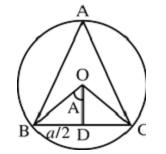# Sine Formula

In any triangle ABC,

a/sinA = b/sin B = c/sinC = 2R

R is the radius of the circumcircle of the triangle ABC.From the right angled triangle BOD,

sinA = BD/R = a/2R

2R sinA = a

a/sinA = 2R